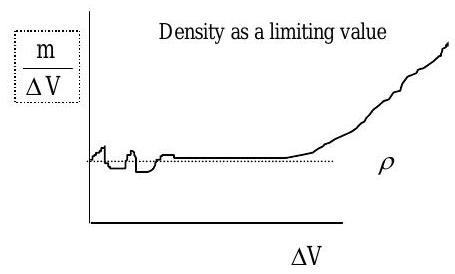# 3.6: Density, Specific Volume, Specific Weight, and Specific Gravity

$$\newcommand{\vecs}{\overset { \rightharpoonup} {\mathbf{#1}} }$$ $$\newcommand{\vecd}{\overset{-\!-\!\rightharpoonup}{\vphantom{a}\smash {#1}}}$$$$\newcommand{\id}{\mathrm{id}}$$ $$\newcommand{\Span}{\mathrm{span}}$$ $$\newcommand{\kernel}{\mathrm{null}\,}$$ $$\newcommand{\range}{\mathrm{range}\,}$$ $$\newcommand{\RealPart}{\mathrm{Re}}$$ $$\newcommand{\ImaginaryPart}{\mathrm{Im}}$$ $$\newcommand{\Argument}{\mathrm{Arg}}$$ $$\newcommand{\norm}{\| #1 \|}$$ $$\newcommand{\inner}{\langle #1, #2 \rangle}$$ $$\newcommand{\Span}{\mathrm{span}}$$ $$\newcommand{\id}{\mathrm{id}}$$ $$\newcommand{\Span}{\mathrm{span}}$$ $$\newcommand{\kernel}{\mathrm{null}\,}$$ $$\newcommand{\range}{\mathrm{range}\,}$$ $$\newcommand{\RealPart}{\mathrm{Re}}$$ $$\newcommand{\ImaginaryPart}{\mathrm{Im}}$$ $$\newcommand{\Argument}{\mathrm{Arg}}$$ $$\newcommand{\norm}{\| #1 \|}$$ $$\newcommand{\inner}{\langle #1, #2 \rangle}$$ $$\newcommand{\Span}{\mathrm{span}}$$

As we have demonstrated earlier, the mass of a system is an extensive property and as such it depends upon the extent of the system. Recall that for a specified system, the mass of the system can be calculated from this general relationship: $m_{sys}=\int_{V_{sys}} \rho \ dV \nonumber$ It would be impossible to tabulate a handbook of masses for all possible combinations of substances and systems, but it is feasible to tabulate or provide models for the density. Once this information is known, this equation can be used to calculate the mass of the system.

## Density and Specific Volume

The density of a substance is usually found tabulated in handbooks as a function of the substance and its state (i.e. its temperature and pressure). The density $$\rho$$ of a substance is the mass per unit volume and typically has units of $$\mathrm{kg} / \mathrm{m}^{3}$$ or $$\mathrm{lbm} / \mathrm{ft}^{3}$$.

Mathematically, the density at a point in space can be thought of as the limiting value of the mass per unit volume within a small cube of volume as the volume shrinks towards zero: $\rho = \lim_{\Delta V \rightarrow 0} \frac{m}{\Delta V} \nonumber$ Imagine, if you will, that as the cube of volume becomes smaller and smaller the density approaches some limiting value (See Figure $$\PageIndex{1}$$). At some point the volume of the cube becomes small enough that the number of molecules within the volume begins to fluctuate with time. Once this point is reached, the idea of a statistically significant average value (or limit) makes no sense.Figure $$\PageIndex{1}$$: Density as a limiting value.

In this course we are restricting ourselves to conditions under which this type of statistically significant average is possible. This is called the continuum assumption. Sometimes this viewpoint is also called a macroscopic viewpoint because it only addresses macroscopic variables like pressure, temperature, density, etc. One of the distinct advantages of a macroscopic or continuum viewpoint is that we do not have to explicitly consider the microscopic behavior of atoms and molecules. In this course, we will only be considering continuums and a macroscopic viewpoint. Sometimes we may find it useful to take a microscopic viewpoint to help us understand some phenomena. Although in most industrial situations, the continuum assumption is appropriate, there are many important situations where the continuum assumption breaks down. These are typically conditions with very low densities, e.g. vacuum conditions, conditions in space near the edge of the earth's atmosphere.

For some substances it is not the density but the specific volume that is tabulated. The specific volume $$v$$ is the volume per unit mass. It is typically given the lower-case "v" as its symbol and can be related to the density by the equation: $v=\frac{1}{\rho} \nonumber$ The dimensions for specific volume are $$[\mathrm{L}]^{3} / [\mathrm{M}]$$, and typical units for specific volume are $$\mathrm{m}^{3} / \mathrm{kg}$$ or $$\mathrm{ft}^{3} / \mathrm{lbm}$$.

As one might expect, there is a molar density defined as the moles per unit volume and given the symbol $$\bar{\rho}$$, where the overbar indicates that this is a mole-based quantity. A molar specific volume is also defined similarly as the volume per unit mole and is given the symbol $$\bar{v}$$.

What would be the units for these two molar quantities in SI and in the American Engineering System?

## Specific Weight

The specific weight of a substance is the weight per unit volume. Specific weight is usually given the symbol $$\gamma$$ ("gamma") and can be calculated as the product of density times the local acceleration of gravity: $\gamma=\rho g \nonumber$ Any values of specific weight you find listed in a table have, of necessity, assumed a value for the local acceleration of gravity. Unless noted otherwise, tabulated specific weight values are based on the standard value for the acceleration of gravity at sea level, $$9.80665 \mathrm{~m} / \mathrm{s}$$ or $$32.174 \mathrm{ft} / \mathrm{s}$$. Typical units for specific weight are $$\mathrm{N} / \mathrm{m}^{3}$$ or $$\mathrm{lbf} / \mathrm{ft}^{3}$$.

## Specific Gravity

In many applications, it is important to know the density or specific weight of a substance relative to that of a reference substance.. The specific gravity $$( \boldsymbol{SG} )$$ of a substance is the ratio of the density of the substance at the specified conditions to the density of a reference substance: $S G=\frac{\rho}{\rho_{\text{ref}}} \nonumber$ For liquids and solids, the reference substance is water at $$4^{\circ} \mathrm{C}$$ where the density of water is $$1000 \mathrm{~kg} / \mathrm{m}^{3}$$ or $$1.940 \ \mathrm{slug} / \mathrm{ft}^{3}$$. For gases, the reference substance is air at a specified temperature and pressure, say $$25^{\circ} \mathrm{C}$$ and 1 atm. Under these conditions, air can be treated as an ideal gas.

3.6: Density, Specific Volume, Specific Weight, and Specific Gravity is shared under a CC BY-NC-SA 4.0 license and was authored, remixed, and/or curated by LibreTexts.